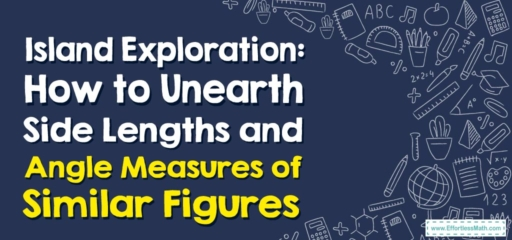# Island Exploration: How to Unearth Side Lengths and Angle Measures of Similar Figures

Ahoy, fellow explorers!

For our next adventure, we're setting sail to an island filled with the mystique of similar figures. Prepare your compasses and protractors, and let's dive into the exciting world of geometry!## 1. Mapping Out the Island: Understanding Similar Figures

Before we embark, let’s chart our map:

• Similar Figures: These are figures that are the same shape but not necessarily the same size. Their side lengths are proportional, and their corresponding angles are equal.

## 2. The Treasure Hunt: Finding Side Lengths and Angle Measures of Similar Figures

Equipped with our understanding of similar figures, let’s uncover the treasures of geometry!

### Explorer’s Guide: Discovering Side Lengths and Angle Measures of Similar Figures

#### Step 1: Identify Similar Figures

First, determine if you’re dealing with similar figures. This will be the case if their corresponding angles are equal and their sides are in proportion.

#### Step 2: Use the Similarity Criteria

Once we’ve identified similar figures, we can use the similarity criteria to find unknown side lengths. The ratios of corresponding sides in similar figures are equal.

#### Step 3: Solve for the Unknown

Finally, we solve for the unknown value using these ratios.

For instance, if we have two similar rectangles, and the sides of the first one are $$6\ cm$$ and $$9\ cm$$, while one side of the second one is $$12\ cm$$, what is the length of the other side?

1. Identify Similar Figures: Both rectangles are similar.
2. Use the Similarity Criteria: The sides are proportional, so $$\frac{6}{9} = \frac{12}{x}$$.
3. Solve for the Unknown: Cross-multiply and solve for $$x$$, giving us $$x = 18\ cm$$.

And just like that, we’ve successfully navigated the mysterious world of similar figures! Remember, fellow explorers, geometry is a treasure map, guiding us to the wonders of the mathematical world. Until our next exploration, stay curious!

### What people say about "Island Exploration: How to Unearth Side Lengths and Angle Measures of Similar Figures - Effortless Math: We Help Students Learn to LOVE Mathematics"?

No one replied yet.

X
30% OFF

Limited time only!

Save Over 30%

SAVE $5 It was$16.99 now it is \$11.99